Map Projections

The Earth is a spheroid - it is round like a ball or sphere but flattened by slightly by the centrifugal force of rotation. The Earth has a circumference of around 24,900 miles, but is around 27 miles wider than it is tall.

The Earth exists in three-dimensions but, other than globes, most representations of the earth, such as maps, are two dimensional. A projection is a set of mathematical transformations used to represent the three-dimensional world in two dimensions.

Datums

One key element of projection is the datum that is used to represent the world.

The lumpy surface of the earth is approximated with a simplified representation called a geoid. Then a smooth mathematical ellipsoid representation of the geoid that is used as the reference for latitude and longitude degrees. This reference system is caled a geodetic datum.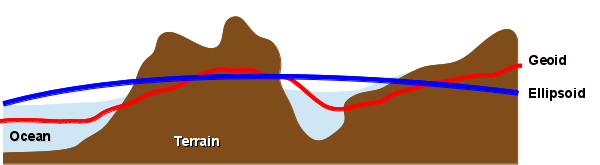Relationship Between Terrain, an Datum, and an Ellipsoid

The science of creating geoids is called geodesy or geodetics. Geodetic engineers measure three fundamental properties of the earth:

• Geometric shape
• Orientation in space
• Gravity field

Because these properties are constantly changing (albeit in often subtle ways), geodetic engineers always have something to do in support of the earth sciences.

With contemporary geospatial data, the World Geodetic System from 1984 (WGS 84) used by the Global Positioning System (GPS) is the datum you will encounter most frequently. Datums you may also encounter (especially with older data) include the North American Datums from 1927 (NAD27) and 1983 (NAD83), although GIS software will usually handle transformation from those datums automatically with no additional attention needed on your part.

Distortion

The transformation (projection) of three-dimensional reality to a two-dimensional representation always involves distortion of at least three of four properties of reality:

• Area
• Shape
• Direction
• Distance

The ultimate purpose of a particular visualization (map) determines which of these properties to preserve:

• Equal-area or equiareal projections preserve area. This is primarily important for general-interest maps where the size of areas (such as countries) is used to judge their relative importance
• Equidistant projections preserve distance and are useful for situations such as transportation or property boundaries
• Conformal projections preserve angles (and, therefore, shape or direction) and are useful for navigation. Note that conformal maps distort distance, so even though they can be used to point you in the right direction, that may not ultimately be the shortest path, especially over long distances
• Compromise projections attempt to balance the distortion of all four properties in a way that makes maps useful for specific purposes

To observe the distortions associated with a particular projection, Tissot's Indicatrix is a map of circles regularly spaced across the surface of the area covered by a map. This makes it possible to observe the different types of distortion at different locations on the map. Tissot's Indicatrix is used in the projection examples below.

Coordinate Systems

While the ubiquity of GPS has led to the popularity of specifying locations with coordinates using WGS 84 longitudes and latitudes, there are a wide variety of coordinate systems. While locations are still represented with pairs of numbers (and sometimes a third number for elevation), the meaning of those numbers and the range of possible values differ depending on which coordinate system you are using.

There are two broad types of coordinate systems:

• Geographic coordinate systems indicate where something is on the surface of the planet
• Projected coordinate systems indicate where something is on a map or other representation of the surface of the planet

While we live in a three-dimensional world, our experience of and interaction with the world at human scale is often perceived of and represented in two dimensions. We think of the distances between locations and objects as lines rather than degrees curved over the surface of the planet.

Accordingly, coordinate systems used by surveyors and the military often express coordinates in meters or feet, permitting quick calculation of distance.

Similarly, these coordinate systems are used by GIS software to indicate where to draw features on the screen when using a specific projection. Although the software will display these coordinate numbers, they are not usually that directly useful to the cartographer except when attempting to diagnose problems.

The process of transforming geospatial data from one projection to another is called reprojection. Reprojection can introduce errors into the data because of limitations in the accuracy of the way numbers are represented in digital computers and the differing characteristics of different projections. Caution must be exercised in choosing projections that are appropriate to the geographic area of the data (e.g. choosing the correct UTM or State Plane Coordinate System zone). When working with older data, consideration must be given to the datum upon which the projection and coordinate system are based.

With raster data that already represents data as a grid, the projection is locked into the data. It is possible to change the projection of a raster by overlaying the grid for a new projection on top of the old grid and taking the values from the old grid at the locations of the new grid. This process is called resampling. While there are mathematical techniques for making the new grid match the old grid as much as possible (interpolation), resampling always adds errors to raster data and should be avoided whenever possible.

Projection Types

The process of map projection often goes through the imagined step of wrapping a flat map around or thorough an area. This wrapped map is called a developable surface.

While there are hundreds of specific projections, there are a handful of general types associated with different types of developable surfaces that are the basis for those specific projections.

To balance distortions, some projections are compromise projections that incorporate complex transformations from the simple, fundamental types of projections given below.

There are three basic types of developable surface: the plane, the cylinder and the cone.

Cylindrical Projections

These projections are equivalent to wrapping the map as a cylinder around the earth.

This wrapping can be around the equator, or around the poles (transverse cylindrical).

Conic Projections

These projections imagine the map wrapped as a cone that intersects the surface of the earth in one or two places, which are called standard parallels.

Azimuthal

These projections treat the map as a flat surface or plane. They are commonly used for maps of the north or south pole.

Unprojected Longitude / Latitude

Since longitude and latitude represent x/y coordinates, they can be directly graphed on a grid, and this is the default when you open a feature set in ArcMap. However, because longitudes are closer together the further the further north you go from the equator, the shapes of areas are flattened

Unprojected longitude and latitude is useful for diagnosing problems with data, but should not be used in any professional presentation, as it implies carelessness or ignorance of cartographic principles and standards.

Identifying Projections

While some common projections have names that are generally understood (like spherical mercator), a name is not usually enough to indicate what specific projection you want to use. For example, although the Mercator is a well known general type of projection, different Mercator projections have different parameters depending on how they wrap the world in different ways (transverse, oblique) at different places (central meridian) and using different coordinate units.

An exhaustive list of different coordinate system transformation identifiers is available at SpatialReference.org

There are three common conventions use to formally identify specific projections.

The Well-Known ID (WKID) number is an ID number commonly used to reference a projection. The European Petroleum Survey Group (EPSG) maintains a numbered registry of coordinate reference systems and transformations. One issue with this numbering system is that there is not a clear structure to the numbering scheme, the same projection can have different numbers, and there are different registries or authorities that use different numbers. EPSG numbers are given below in the list of suggested projections. For example, a commonly-used WGS84 / World Mercator projection is EPSG:3395

PROJ.4 is a software library used to transform between coordinate systems. There is a standard set of PROJ.4 strings used to formally specify types of projections and the parameters used with a specific projection. For example, the PROJ.4 string for the EPSG 3395 World Mercator projection (EPSG 3395) is:

+proj=merc +lon_0=0 +k=1 +x_0=0 +y_0=0 +datum=WGS84 +units=m +no_defs

Well-Known Text (WKT) convention is an international standard (ISO 19162) for specifying coordinate transformations. This format is much more detailed than PROJ.4 and can be difficult to read. For example, the WKT for the EPSG 3395 World Mercator projection is:

PROJCS["WGS 84 / World Mercator",
GEOGCS["WGS 84",
DATUM["WGS_1984",
SPHEROID["WGS 84",6378137,298.257223563,
AUTHORITY["EPSG","7030"]],
AUTHORITY["EPSG","6326"]],
PRIMEM["Greenwich",0,
AUTHORITY["EPSG","8901"]],
UNIT["degree",0.01745329251994328,
AUTHORITY["EPSG","9122"]],
AUTHORITY["EPSG","4326"]],
UNIT["metre",1,
AUTHORITY["EPSG","9001"]],
PROJECTION["Mercator_1SP"],
PARAMETER["central_meridian",0],
PARAMETER["scale_factor",1],
PARAMETER["false_easting",0],
PARAMETER["false_northing",0],
AUTHORITY["EPSG","3395"],
AXIS["Easting",EAST],
AXIS["Northing",NORTH]]

Suggested Projections

There are dozens of different types of projections. When you consider that many of those projections have parameters that can be varied depending on the part of the earth you wish to focus on, the number of possible projections is infinite.

However, there are a much more limited number of projections that are commonly used. The following are some North-America-centric suggestions that can give you a good starting point in seeking an appropriate projection for your map.

World Maps

Mercator is one of the oldest and most commonly seen projections. It is a cylindrical projection devised by the Flemish cartographer Gerardus Mercator (1512-1594) during the great age of European exploration, and as such it is conformal (preserves direction) in order to facilitate naval navigation.

The primary disadvantage of the Mercator is that it makes areas close to the poles (such as Greenland) look much larger than they really are.

A commonly used Mercator projection covering the entire world is EPSG 3395: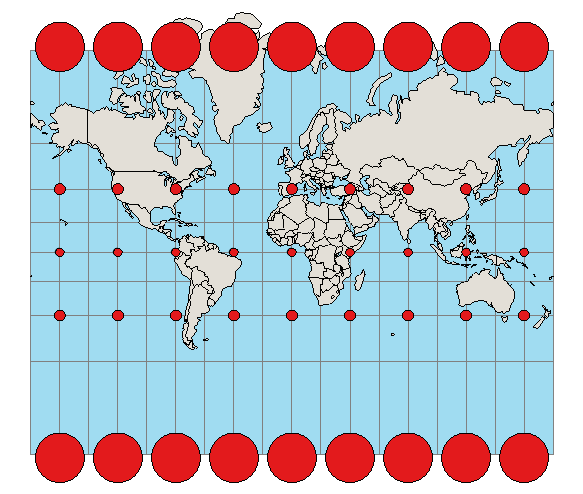Spherical Mercator is a variation of the Mercator projection that mathematically represents the world as a sphere rather than an ellipsoid to make calculations easier. This is the projection used by almost all web maps. Because the world slightly flattened, this spherical representation causes the map to be inaccurate when mapping large areas closer to the poles. Spherical Mercator has had a variety of ID numbers, but EPSG: 3857 is the current standard.

The Robinson projection is a compromise projection developed by Arthur Robinson in 1964. The Robinson projection solved the "Greenland Problem" by representing the country closer to its actual size than the Mercator projection. The Robinson projection is considered by many to be visually appealing and in 1988 was adopted by the National Geographic Society for some of its world maps in place of the older Van der Grinten projection. The World Robinson projection is EPSG: 54030.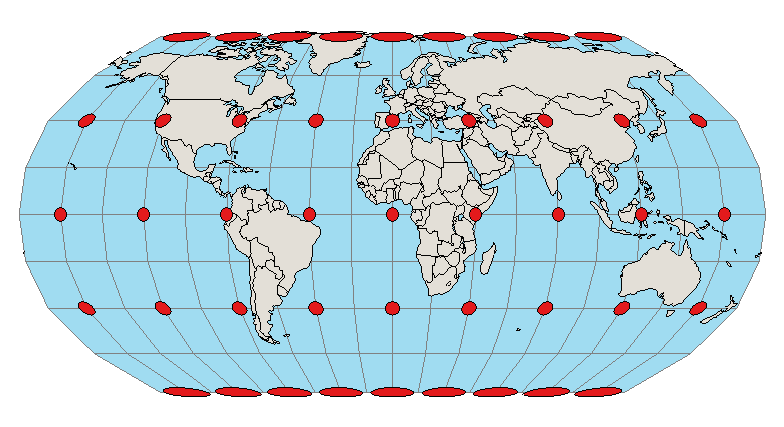Winkel Tripel is a compromise projection devised by Oswald Winkel in 1921 that strives to represent the whole world while minimizing distortion of area, direction and distance (tripel means three in German). This is the projection used for maps by the National Geographic Society and many educational publishers. The Winkel Tripel does not have an EPSG number.

McArthur's Universal Corrective Map is one of a number of alternative projections intended to provoke viewers to critically examine assumptions about maps. It is a variant on a Mercator projection by Australian cartographer Stuart McArthur that debuted in 1979 with a reversal of north and south.

There is, of course, no geological or astrophysical reason why north should be up, and the reversal of the poles asks us to call into question the biases inherent to a traditional north-up orientation.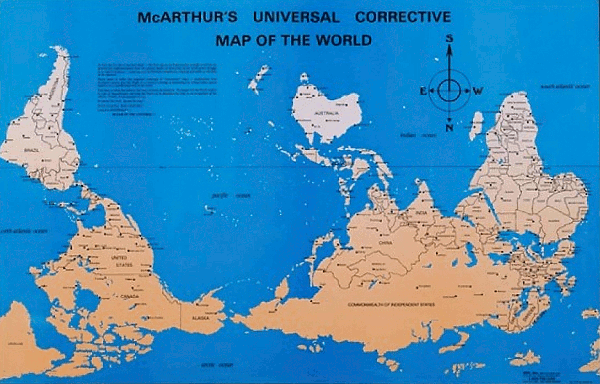Large Country and Continent Maps

Albers Equal Area Conic is a common projection for maps of the continental United States that has historically been used by the United States Geological Society (USGS). It is a conic projection with two standard parallels that intersect the earth, and it preserves area while slightly distorting shape. An Albers Equal Area Conic projection commonly used for maps of North America is EPSG:102008.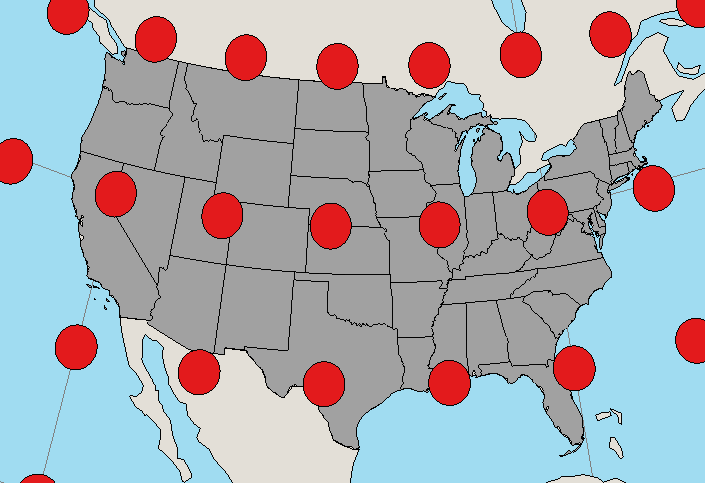Albers Equal Area Conic Projection for North America

Lambert Conformal Conic is another conic projection commonly used for the continental United States. While it distorts area, it preserves shapes better, and since it preserves angles (conformal) it is commonly used for aeronautical charts since a straight line on the map approximates the shortest-path great-circle distance between two points. A Lambert Conformal Conic projection commonly used for US maps with Texas in the center is EPSG:3082.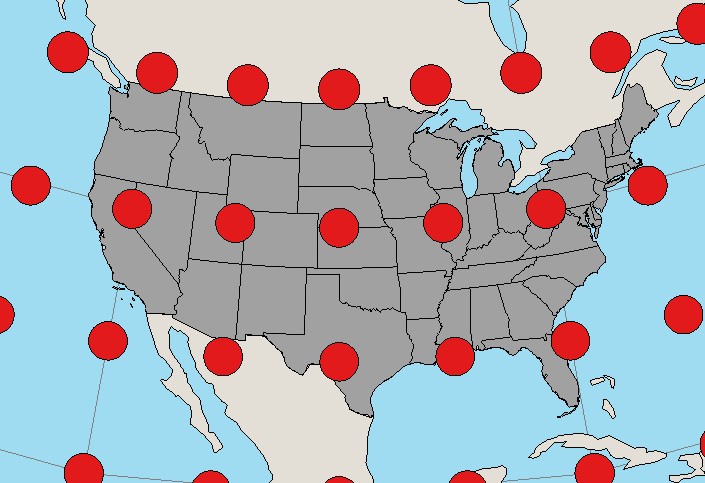Lambert Conformal Conic Projection for North America

Local Maps

While projections for world or country maps are primarily focused on presentation or navigation, projections at the local level are more important for the ability to accurately find specific locations and measure distances, such as when surveying property lines or engaging in military activity.

The State Plane Coordinate System is a set of projections commonly used by local and regional governments. They are based on Lambert Conic or Transverse Mercator projections that are conformal and, therefore, can be conveniently used for calculating distance.

There are 124 different zones in different parts of the US. They can be oriented with the long side of the zone going east-west (for example, Iowa's zones are conic) or north-south (Illinois' zones are transverse Mercator).

The small size of these zones means that distortion of distance can be greatly minimized to 1 part in 10,000. You can measure a distance of two miles within around a foot of accuracy

There are a number of different versions of the State Plane Coordinate System that have been used over the past century (NAD27, NAD83, HARN, CORS96, NSRS2007), and you should choose the version most commonly used in your organization. If you have a choice and high accuracy is not an issue (such as for simple cartographic representations), the easiest option is the NAD83 variant.

ArcMap allows you to search and select from a variety of state plane coordinate systems in the coordinate reference system dialog.

Universal Transverse Mercator (UTM) is a coordinate system based on the Mercator projection and used as an alternative to latitude and longitude. It was devised by the US Army Corps of Engineeers in the 1940s to make land navigation easier and is still used as the basis for the military's Military Grid Reference System.

The world is divided into zones and the coordinates within each zone are specified in meters. Because the projection preserves distance (conformal) and the distortions of distance within small areas on a Mercator are minimal, distance calculations are easier when conducting military operations within fairly small areas.

Projections in ArcMap

1. Identifying the coordinate system of a data layer (0:15)
2. Geographic coordinate systems vs projected coordinate systems (0:40)
3. Global projections: World Mercator and Robinson (1:40)
4. USA projections: World Mercator, North American Albers Equal Area Conic, Lambert Conformal Conic (2:10)
5. State Plane Coordinate System Zones (2:45)
6. Displaying a subset of data: definition queries (3:15)
7. Local projections and the State Plane Coordinate System (3:45)
8. Setting missing coordinate system information (5:20)

Map Projections in R

Projections in R are handled by the RGDAL library and are usually specified using PROJ.4 strings that can be given as parameters to the CRS() function, and in turn used when either creating spatial objects from coordinates, or transforming the coordinates to another coordinate reference system.

The data for this example is in 2017-state-data.zip.

To get a proj4 string For R, you can search SpatialReference.org.

library(rgdal)

# Load the shapefile
states = readOGR(".", "2017-state-data", stringsAsFactors=F)

# Select only the contiguous 48 states
states = states[((states\$ST != "AK") & (states\$ST != "HI")),]

# Transform to Albers Equal Area Conic to make cartographically proper
usa_albers = CRS("+proj=aea +lat_1=29.5 +lat_2=45.5 +lat_0=37.5 +lon_0=-96 +x_0=0 +y_0=0
+ellps=GRS80 +datum=NAD83 +units=m +no_defs")
states = spTransform(states, usa_albers)

# Plot a red-state/blue-state choropleth
colors = ifelse(states\$WIN2012 == "Obama", "navy", "red")
plot(states, col = colors)

legend(x="bottomleft", legend=c("Obama","Romney"),
title="2012 US Presidential Election",
pch=15, col=c("navy","red"))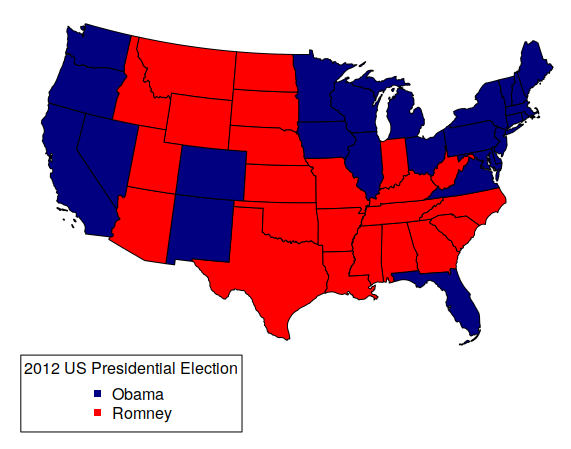Example US Choropleth Using an Albers Equal Area Conic Projection

The following is a list of variables with the PROJ.4 strings used in the examples of suggested projections above:

# EPSG:4326 Unprojected WGS 84 latitude and longitude
# NEVER USED THIS IN A PRINT MAP
wgs84 = CRS("+proj=longlat +ellps=WGS84 +datum=WGS84 +no_defs")

# EPSG: 3395 World Mercator
world_mercator = CRS("+proj=merc +lon_0=0 +k=1 +x_0=0 +y_0=0 +datum=WGS84 +units=m +no_defs")

# EPSG: 3857 Spherical Mercator
spherical_mercator = CRS("+proj=merc +a=6378137 +b=6378137 +lat_ts=0.0 +lon_0=0.0
+x_0=0.0 +y_0=0 +k=1.0 +units=m +nadgrids=@null +wktext  +no_defs")

# EPSG: 54030 World Robinson
robinson = CRS("+proj=robin +lon_0=0 +x_0=0 +y_0=0 +datum=WGS84 +units=m +no_defs")

# EPSG: 102008 North America Albers Equal Area Conic
usa_albers = CRS("+proj=aea +lat_1=20 +lat_2=60 +lat_0=40 +lon_0=-96 +x_0=0 +y_0=0